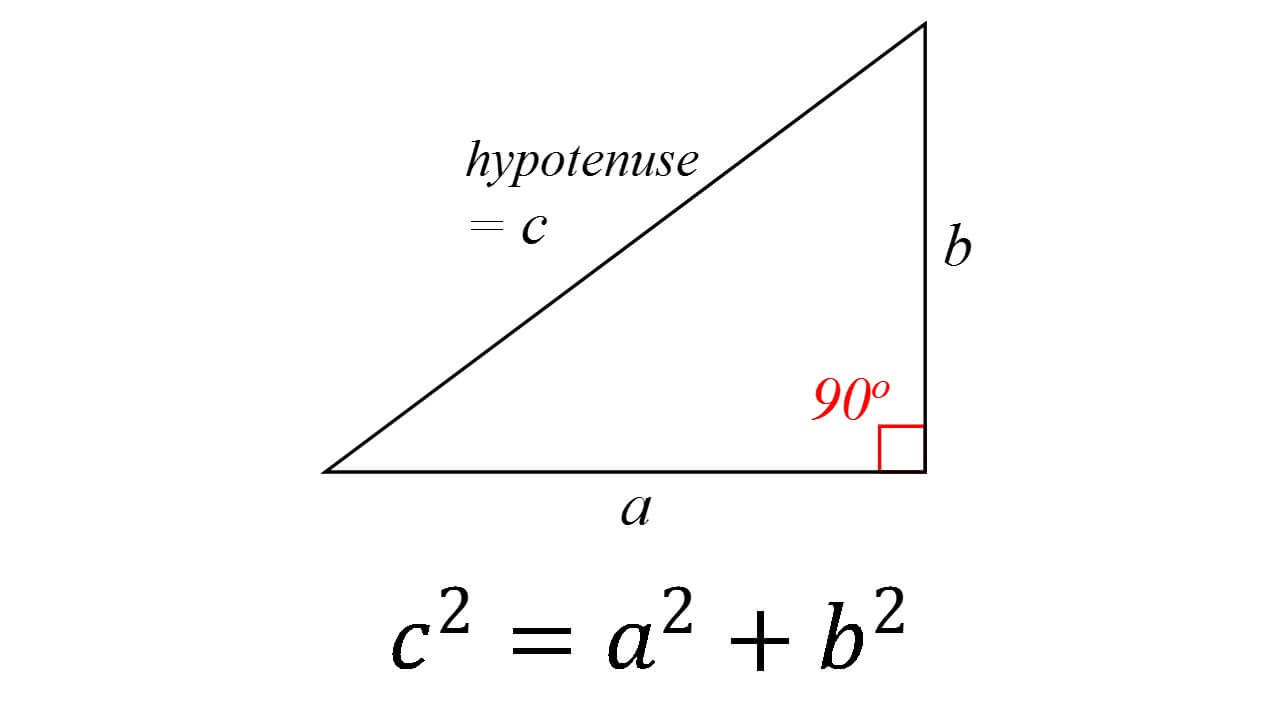# Theory of Pythagoras

The theory of Pythagoras is one of the most relevant mathematical laws of all time thanks to the huge amount of applications that can exist thanks to this important theory.

Pythagoras was the leader of the movement known as Pythagoreanism which, among their major advances in the different fields they studied, had the theory of Pythagoras as one of their pillars, always holding as a principle doctrine that numbers are the primary tool we have to maintain harmony in the known universe.

The theory of Pythagoras, also known as hypotenuse theory, shows us the main relationship that exists within right triangles and has been famous for a very long time. This theory state that when we square the side opposite the right angle, which we call the hypotenuse, we get the same value as if we square each of the remaining sides, known as legs, and add them together.Although there is no documentation that proves that the theory of Pythagoras was Pythagoras himself, there are proofs based on the similarities that exist in triangles that are attributed to this great thinker who lived in the 5th century BC. What we know is that Pythagoras and the Pythagorean School that he founded and directed gave the human race great tools which even today are fundamental in solving problems that may seem simple, but we must recognize all of the prior study that exists to be able to come up with a formula as simple as the formula of the theory of Pythagoras, thanks to which we can shorten our calculations and find the answer much more quickly.

The simplicity of the theory of Pythagoras and the many applications it can be used for make it a mathematical theory that is taught from the first years of school.